# Pulley Lab var pfHeaderImgUrl = '';var pfHeaderTagline = '';var pfdisableClickToDel = 0;var pfHideImages = 0;var pfImageDisplayStyle = 'block';var pfDisablePDF = 0;var pfDisableEmail = 0;var pfDisablePrint = 0;var pfCustomCSS = '';var pfEncodeImages = 0;var pfShowHiddenContent = 0;var pfBtVersion='2';(function(){var js,pf;pf=document.createElement('script');pf.type='text/javascript';pf.src='//cdn.printfriendly.com/printfriendly.js';document.getElementsByTagName('head').appendChild(pf)})();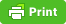Essential Question: What is the relationship between the number of pulleys and the force required to lift the mass?

Essential Question 2: What is the relationship between force required to lift the mass and the length of the rope?

## Site 1: Pulley Lab at Tandftechnology.com ( bit.ly/pulley1 )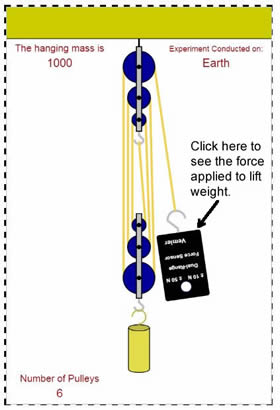Simulation: In this program, you can change the size of the mass and change the number of pulleys. You can also change gravity by changing the planet where you conduct the experiment. Clicking on the vernier probe will show you how much force is being applied to lift the mass.

1. On the image of the pulley, indicate the INPUT FORCE and the OUTPUT FORCE.

2. Change the number of pulleys and fill out the table below.

 Number of Pulleys Input Force 6 5 4 3 2 1

3. Complete this sentence. As you increase the number of pulleys........

4. How could you apply this knowledge to moving furniture?

## Site 2: Pulley at Interactagram.com → bit.ly/pulley1b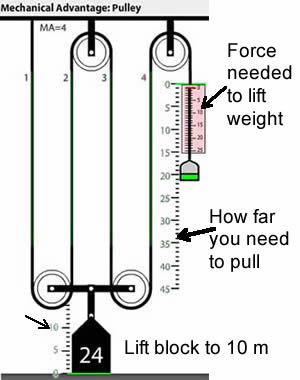In this diagram you are shown a picture of three pulleys, each with the same size weight attached. You can use the handles to lift each block. The image shows you how to take readings on each of your systems to complete the table.

 Number of Pulleys Force needed to lift 24 kg block 10 meters (reading on pink scale) How far you needed to pull the handled down. 4 pulley System 3 pulley System 2 pulley System

6. Read the paragraph at the bottom that explains what mechanical advantage (MA) is. The mechanical advantage is calculated by the following formula:

Load Force (24) divided by Input force (reading on the pink scale)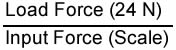Calculate the mechanical advantage for the: (show work)

4 pulley system

3 pulley system

2 pulley system

## Site 3: Pulley Simulation at compassproject.net →bit.ly/pulley3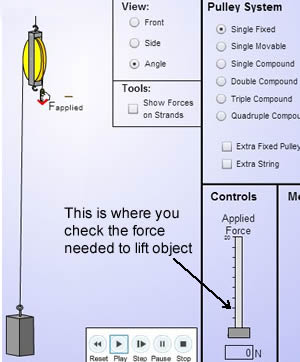Leave the settings as they are, you will just be toggling the buttons under "pulley system" to compare.

7. Run the simulation using a "single fixed" pulley and then a "single movable" pulley.

Which one requires the least amount of applied force? ________________

Which one has the largest "distance pulled? _________________________

Which type of pulley would be best for raising a piano up to a 3rd story window? ______________________

8. Run the simulation using a "single compound" pulley system.

How much applied force is needed to raise the 5N load? _________

Change the load to 10N, how much applied force is needed now? ________

With two pulleys, you need to pull with
a) twice as much force
b) half as much force
c) the same amount of force

9. Change the simulation to "double compound" and leave the load at 10N.

How much applied force is needed to raise the 10N load? _________

Change the Load to 4N (you can just type it in). How much applied force is needed to raise it? ________

Using common sense (and math), what applied force would be needed to lift a weight of 40N?
a) 10    b) 20     c) 30     d) 40

How many pulleys do you think are in this double compound system? a) 1    b) 2    c) 3      d) 4

10. Check the beginning of this handout. There are two essential questions that you were supposed to have answered as you did these investigations. CHOOSE ONE of those questions and write a complete, thoughtful sentence that answers the question.